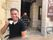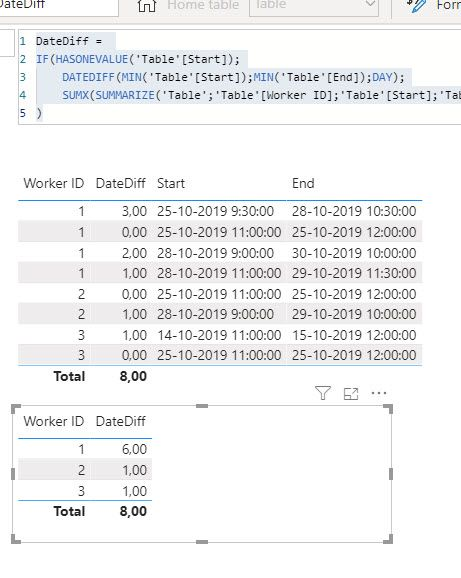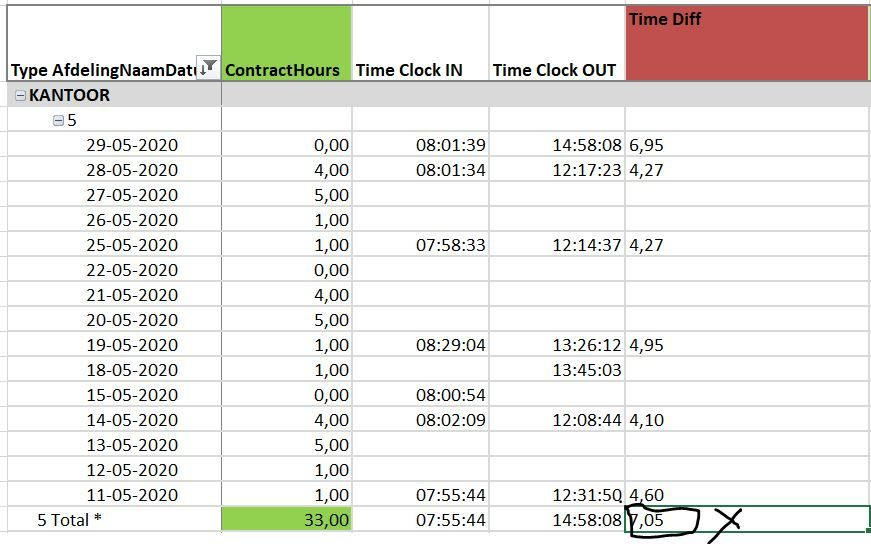cancel
Showing results for
Did you mean:Regular Visitor

## Grand total of datediff not correct

I have a simple statement:

TimeToComplete = DATEDIFF([DateRaised],[DateCompleted],DAY).

I am able to filter by employee and the TimeToComplete column is correct for each of the employee's entries.  However, the Grand Total is equal to the sum of all employees' TimeToComplete.  I need to have the Grand Total be only the sum of the selected employee.  What am I doing wrong?  Thanks.
2 ACCEPTED SOLUTIONSMemorable Member

Hi @kbebow ,

``````DateDiff =
IF(HASONEVALUE('Table'[Start]);
DATEDIFF(MIN('Table'[Start]);MIN('Table'[End]);DAY);
SUMX(SUMMARIZE('Table';'Table'[Worker ID];'Table'[Start];'Table'[End];"Diff"; CALCULATE(DATEDIFF(MIN('Table'[Start]);MIN('Table'[End]);DAY)));[Diff])
)``````

As seen here:File is here

Pls mark as solution if so. Thumbs up for the effort appreciated!

Groet, Steve.

Proud to be a Super User!Memorable Member

Glad I could help, thumbs up  for the effort would be great. Thanks!

Proud to be a Super User!

8 REPLIES 8Super User

please explain what "selected employee" means. Selected where? In a slicer?

What's the data model looking like?Anonymous
Not applicable

Dear,

I have the same issue, please allow me to explain.

From a report with time logs I have created 3 measures
Time Clock IN = calculate(MIN(Raw_data[Time]),FILTER(Raw_data,Raw_data[Gebeurtenis]="AccessOK"&&Raw_data[Ingang gebied]="CLock IN"))
Time Clock OUT = calculate(MAX(Raw_data[Time]),FILTER(Raw_data,Raw_data[Gebeurtenis]="AccessOK"&&Raw_data[Ingang gebied]="Clock OUT UIT"))
Time diff = IFERROR(DATEDIFF([Time Clock IN],[Time Clock OUT],MINUTE)/60,0)
Each single row (date / employee) result for the measure Time worked is correct, only the total or DateToMonth is showing the numbers as expected.

Any help is much appreciated !Super User

you have not specified what you expect the total to show. Should it be the sum of the computed values?  In that case you need to use HASONEVALUE() or ISINSCOPE() to distinguish between individual items in the visual and the totals.

For the totals you would then have to use a SUMX function. Technically you could also use SUMX for the individual items but it would be overkill since it would ony ever sum up one item.Memorable Member

Hi @kbebow ,

``````DateDiff =
IF(HASONEVALUE('Table'[Start]);
DATEDIFF(MIN('Table'[Start]);MIN('Table'[End]);DAY);
SUMX(SUMMARIZE('Table';'Table'[Worker ID];'Table'[Start];'Table'[End];"Diff"; CALCULATE(DATEDIFF(MIN('Table'[Start]);MIN('Table'[End]);DAY)));[Diff])
)``````

As seen here:File is here

Pls mark as solution if so. Thumbs up for the effort appreciated!

Groet, Steve.

Proud to be a Super User!Frequent Visitor

Great, thank you!Regular Visitor

That worked - thank you so much!Memorable Member

Glad I could help, thumbs up  for the effort would be great. Thanks!

Proud to be a Super User!Anonymous
Not applicable

Meantime I figured out  how you can add up measures that are related to time stamps correctly.

1a) Original measure

Time Clock IN = calculate(MIN(Raw_data[Time]),FILTER(Raw_data,Raw_data[Gebeurtenis]="AccessOK"&&Raw_data[Ingang gebied]="CLock IN"))

2a) and then added another measure

Time Clock IN workable=if(countrows(values(DCalendar[Date]))=1,[Time Clock IN],sumx(values(DCalendar[Date]),[Time Clock IN]))

1b) Original measure
Time Clock OUT = calculate(MAX(Raw_data[Time]),FILTER(Raw_data,Raw_data[Gebeurtenis]="AccessOK"&&Raw_data[Ingang gebied]="Clock OUT UIT"))

2b) and then added another measure

Time Clock IN Correct=if(countrows(values(DCalendar[Date]))=1,[Time Clock OUT],sumx(values(DCalendar[Date]),[Time Clock OUT]))

3) Calculate hours worked

Nett time = IFERROR(DATEDIFF([Time Clock IN Correct],[Time Clock OUT Correct],MINUTE)/60,0)

4)a Deviation to calculate overtime hours

Time diff=[Nett Time]-[ContractHours]

4b) and then added another measure

Overtime hours correct= if(countrows(values(DCalendar[Date]))=1,[Time diff],sumx(values(DCalendar[Date]),[Time diff]))

Steps "b" are resulting in correct sum up / grand total.

I am very aware that steps "a" are not necessary to create, as you can integrate them in step b.

It is just to show you the direction how I solved my issue.